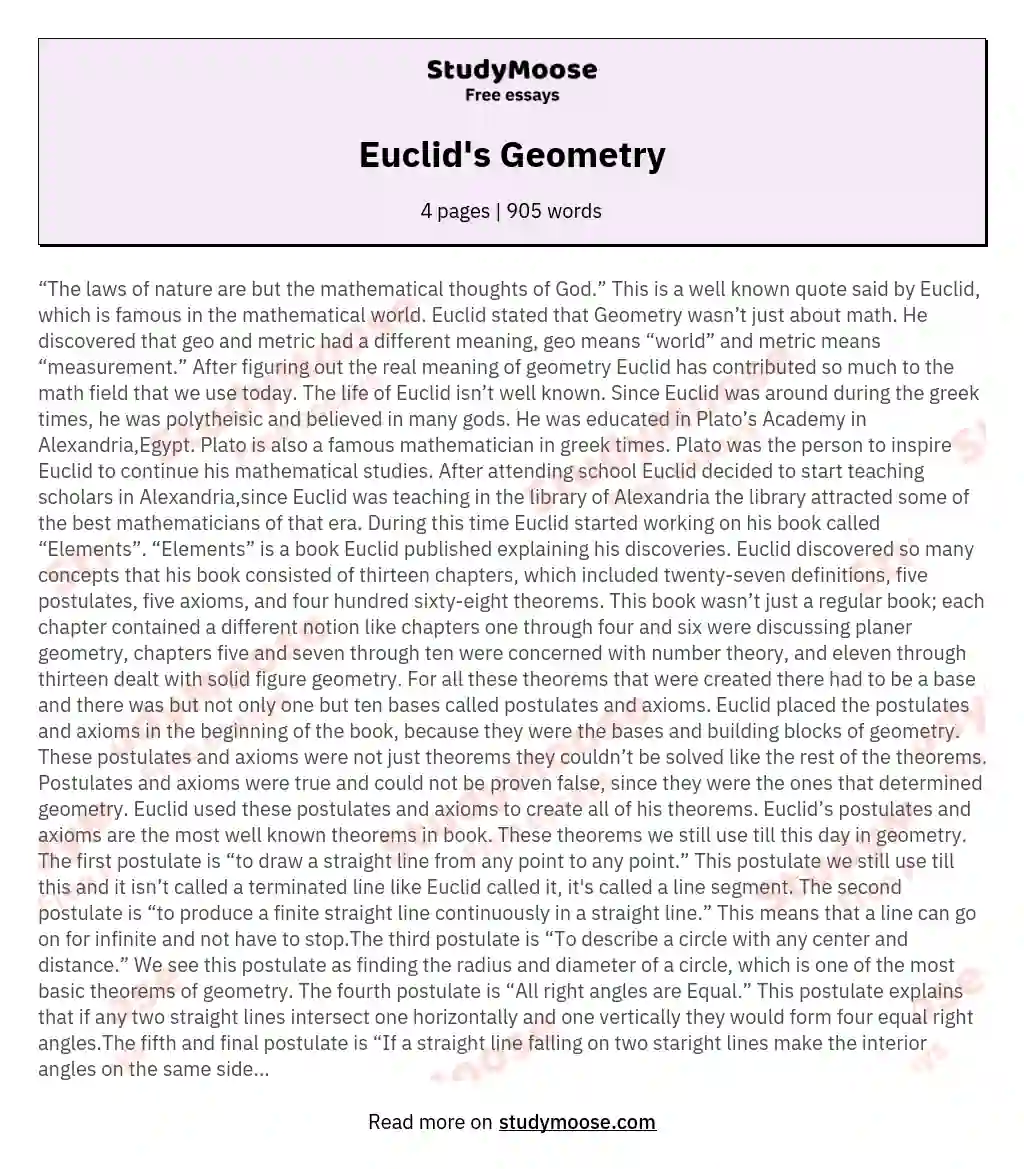# Euclid's Geometry

“The laws of nature are but the mathematical thoughts of God.” This is a well known quote said by Euclid, which is famous in the mathematical world. Euclid stated that Geometry wasn’t just about math. He discovered that geo and metric had a different meaning, geo means “world” and metric means “measurement.” After figuring out the real meaning of geometry Euclid has contributed so much to the math field that we use today.

The life of Euclid isn’t well known.

Since Euclid was around during the greek times, he was polytheisic and believed in many gods. He was educated in Plato’s Academy in Alexandria,Egypt. Plato is also a famous mathematician in greek times. Plato was the person to inspire Euclid to continue his mathematical studies. After attending school Euclid decided to start teaching scholars in Alexandria,since Euclid was teaching in the library of Alexandria the library attracted some of the best mathematicians of that era. During this time Euclid started working on his book called “Elements”.

Get quality help nowBella HamiltonVerified writer

Proficient in: Euclid5 (234)

“ Very organized ,I enjoyed and Loved every bit of our professional interaction ”+84 relevant experts are online

“Elements” is a book Euclid published explaining his discoveries. Euclid discovered so many concepts that his book consisted of thirteen chapters, which included twenty-seven definitions, five postulates, five axioms, and four hundred sixty-eight theorems. This book wasn’t just a regular book; each chapter contained a different notion like chapters one through four and six were discussing planer geometry, chapters five and seven through ten were concerned with number theory, and eleven through thirteen dealt with solid figure geometry. For all these theorems that were created there had to be a base and there was but not only one but ten bases called postulates and axioms.

Get to Know The Price Estimate For Your Paper
Topic
Number of pages
Email Invalid email

You won’t be charged yet!

Euclid placed the postulates and axioms in the beginning of the book, because they were the bases and building blocks of geometry. These postulates and axioms were not just theorems they couldn’t be solved like the rest of the theorems. Postulates and axioms were true and could not be proven false, since they were the ones that determined geometry. Euclid used these postulates and axioms to create all of his theorems.

Euclid’s postulates and axioms are the most well known theorems in book. These theorems we still use till this day in geometry. The first postulate is “to draw a straight line from any point to any point.” This postulate we still use till this and it isn’t called a terminated line like Euclid called it, it’s called a line segment. The second postulate is “to produce a finite straight line continuously in a straight line.” This means that a line can go on for infinite and not have to stop.The third postulate is “To describe a circle with any center and distance.”

We see this postulate as finding the radius and diameter of a circle, which is one of the most basic theorems of geometry. The fourth postulate is “All right angles are Equal.” This postulate explains that if any two straight lines intersect one horizontally and one vertically they would form four equal right angles.The fifth and final postulate is “If a straight line falling on two staright lines make the interior angles on the same side less than two right angles, the two straight lines , if produced indefinitely, meet on the side on which are the angles less than two right angles.”

The postulates aren’t just the only theorems that make up geometry. There are also axioms, axioms can be used in our everyday life. The first axiom is “Things which are equal to the same thing are also equal to one another.” The second axiom is “Things which coincide with one another are equal to one another.” The third axiom is “If equals are added to equals, the wholes are equal.”

The fourth axiom is “If equals be subtracted from equals, the remainders are equal.” The final axioms is “The whole is greater than the part.” All of these axioms are based on equals and these theorems can be used while shopping at a supermarket.

Well all these theorems have really changed geometry and the way we use them today. Euclid’s book was the second most printed book after the bible, that’s how much everyone wanted to get into the teachings of Euclid. Ever since Euclid published his book for two thousand years mathematicians and mathematics classes have used Euclid’s book. Many famous mathematicians who came after Euclid studied his book and proved his theorems to be true. Some of Euclid’s successors include Aristotle and Pythagoras.

Aristotle studied in the library/school Euclid had created and was inspired by Euclid. Pythagoras decided to focus on one of Euclid’s proofs and proved it true, with a theorem known today as the Pythagorean theorem.

Euclid had a mathematical goal to contribute to his time. He not only bestowed the book to the people of his era but to us today. Clearly, we can see that Euclid really did change and grow the mathematical world and made it clearer for us to understand.

## References

1. http://www.math.brown.edu/~banchoff/Beyond3d/chapter9/section01.html
2. http://www.math.wichita.edu/history/Men/euclid.html
3. http://mathshistory.st-andrews.ac.uk/Biographies/Euclid.html
4. https://mathcs.clarku.edu/~djoyce/java/elements/toc.html
5. https://www.friesian.com/space.htm
6. https://mathigon.org/course/euclidean-geometry/axioms
7. https://www.britannica.com/science/Euclidean-geometry
8. https://libquotes.com/euclid/quote/lbx5i0d